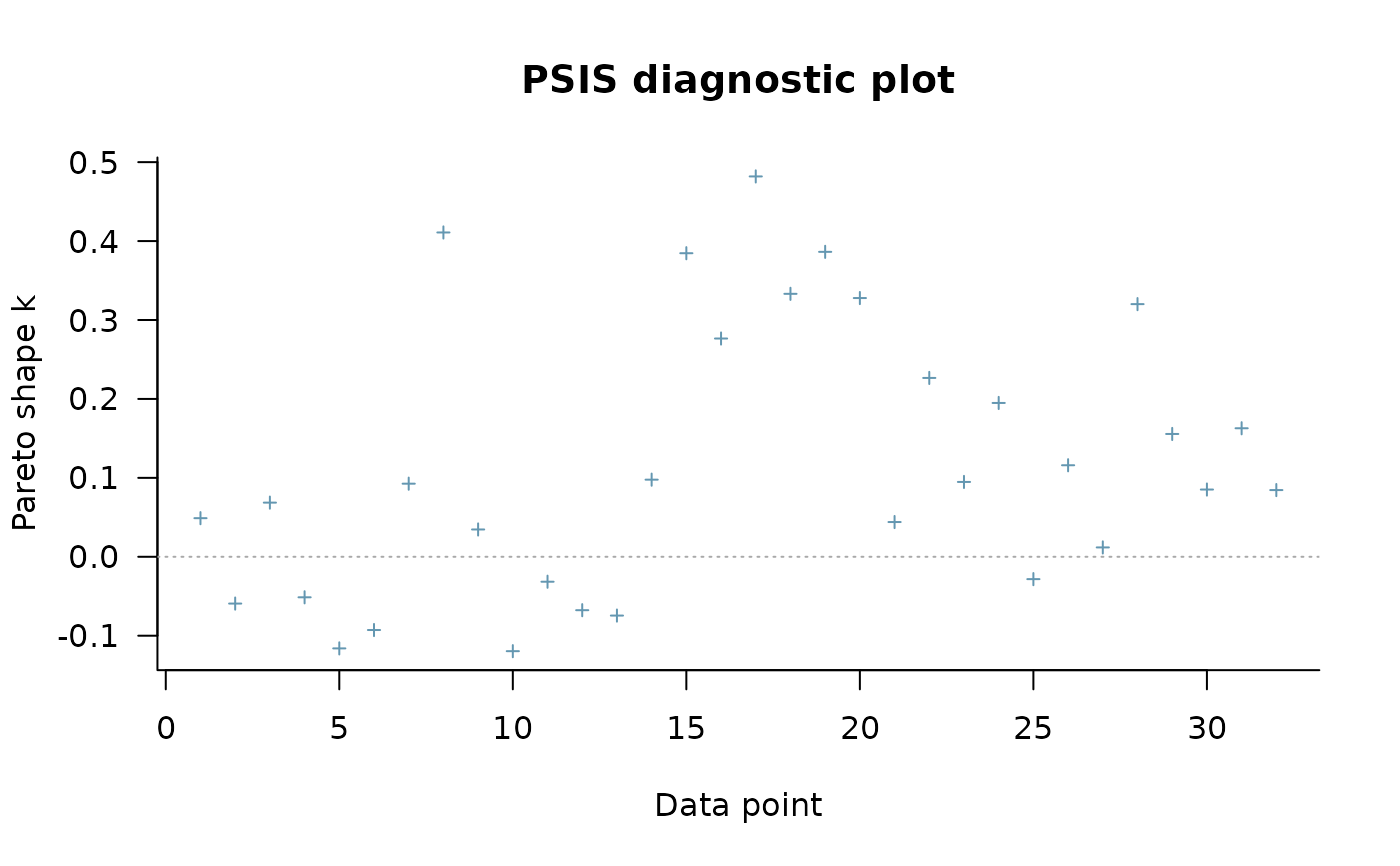Implementation of Pareto smoothed importance sampling (PSIS), a method for stabilizing importance ratios. The version of PSIS implemented here corresponds to the algorithm presented in Vehtari, Simpson, Gelman, Yao, and Gabry (2019). For PSIS diagnostics see the pareto-k-diagnostic page.

psis(log_ratios, ...)

# S3 method for array
psis(log_ratios, ..., r_eff = NULL, cores = getOption("mc.cores", 1))

# S3 method for matrix
psis(log_ratios, ..., r_eff = NULL, cores = getOption("mc.cores", 1))

# S3 method for default
psis(log_ratios, ..., r_eff = NULL)

is.psis(x)

is.sis(x)

is.tis(x)

## Arguments

log_ratios An array, matrix, or vector of importance ratios on the log scale (for PSIS-LOO these are negative log-likelihood values). See the Methods (by class) section below for a detailed description of how to specify the inputs for each method. Arguments passed on to the various methods. Vector of relative effective sample size estimates containing one element per observation. The values provided should be the relative effective sample sizes of 1/exp(log_ratios) (i.e., 1/ratios). This is related to the relative efficiency of estimating the normalizing term in self-normalizing importance sampling. If r_eff is not provided then the reported PSIS effective sample sizes and Monte Carlo error estimates will be over-optimistic. See the relative_eff() helper function for computing r_eff. If using psis with draws of the log_ratios not obtained from MCMC then the warning message thrown when not specifying r_eff can be disabled by setting r_eff to NA. The number of cores to use for parallelization. This defaults to the option mc.cores which can be set for an entire R session by options(mc.cores = NUMBER). The old option loo.cores is now deprecated but will be given precedence over mc.cores until loo.cores is removed in a future release. As of version 2.0.0 the default is now 1 core if mc.cores is not set, but we recommend using as many (or close to as many) cores as possible. Note for Windows 10 users: it is strongly recommended to avoid using the .Rprofile file to set mc.cores (using the cores argument or setting mc.cores interactively or in a script is fine). For is.psis(), an object to check.

## Value

The psis() methods return an object of class "psis", which is a named list with the following components:

log_weights

Vector or matrix of smoothed (and truncated) but unnormalized log weights. To get normalized weights use the weights() method provided for objects of class "psis".

diagnostics

A named list containing two vectors:

• pareto_k: Estimates of the shape parameter $$k$$ of the generalized Pareto distribution. See the pareto-k-diagnostic page for details.

• n_eff: PSIS effective sample size estimates.

Objects of class "psis" also have the following attributes:

norm_const_log

Vector of precomputed values of colLogSumExps(log_weights) that are used internally by the weights method to normalize the log weights.

tail_len

Vector of tail lengths used for fitting the generalized Pareto distribution.

r_eff

If specified, the user's r_eff argument.

dims

Integer vector of length 2 containing S (posterior sample size) and N (number of observations).

method

Method used for importance sampling, here psis.

## Methods (by class)

• array: An $$I$$ by $$C$$ by $$N$$ array, where $$I$$ is the number of MCMC iterations per chain, $$C$$ is the number of chains, and $$N$$ is the number of data points.

• matrix: An $$S$$ by $$N$$ matrix, where $$S$$ is the size of the posterior sample (with all chains merged) and $$N$$ is the number of data points.

• default: A vector of length $$S$$ (posterior sample size).

Vehtari, A., Gelman, A., and Gabry, J. (2017a). Practical Bayesian model evaluation using leave-one-out cross-validation and WAIC. Statistics and Computing. 27(5), 1413--1432. doi:10.1007/s11222-016-9696-4 (journal version, preprint arXiv:1507.04544).

Vehtari, A., Simpson, D., Gelman, A., Yao, Y., and Gabry, J. (2019). Pareto smoothed importance sampling. preprint arXiv:1507.02646

## See also

• loo() for approximate LOO-CV using PSIS.

• pareto-k-diagnostic for PSIS diagnostics.

## Examples

log_ratios <- -1 * example_loglik_array()
r_eff <- relative_eff(exp(-log_ratios))
psis_result <- psis(log_ratios, r_eff = r_eff)
str(psis_result)#> List of 2
#>  $log_weights: num [1:1000, 1:32] 2.37 2.12 2.24 2.41 2.25 ... #>$ diagnostics:List of 2
#>   ..$pareto_k: num [1:32] 0.0447 -0.0593 0.0696 -0.052 -0.1159 ... #> ..$ n_eff   : num [1:32] 901 923 929 896 895 ...
#>  - attr(*, "norm_const_log")= num [1:32] 9.28 9.04 9.25 9.09 9 ...
#>  - attr(*, "tail_len")= num [1:32] 99 98 97 100 100 102 99 100 103 98 ...
#>  - attr(*, "r_eff")= num [1:32] 0.933 0.939 0.968 0.913 0.911 ...
#>  - attr(*, "dims")= int [1:2] 1000 32
#>  - attr(*, "method")= chr "psis"
#>  - attr(*, "class")= chr [1:3] "psis" "importance_sampling" "list"plot(psis_result)# extract smoothed weights
lw <- weights(psis_result) # default args are log=TRUE, normalize=TRUE
ulw <- weights(psis_result, normalize=FALSE) # unnormalized log-weights

w <- weights(psis_result, log=FALSE) # normalized weights (not log-weights)
uw <- weights(psis_result, log=FALSE, normalize = FALSE) # unnormalized weights